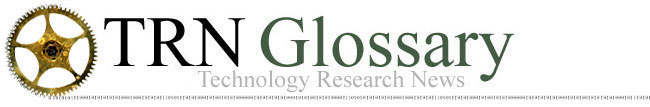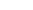A   B   C   D   E   F   G   H   I   J   K   L   M   N   O   P   Q   R   S   T   U   V   W   X   Y   Z

Bayes' Theorem: A formula for calculating conditional probabilities or recalculating probabilities based on additional information. Bayes' Theorem is used for inferential and predictive reasoning in Artificial Intelligence and predictive routing in Networking.

Binary: The base 2 number system, where all numbers are represented by combinations of the digits 0 and 1. Computers use binary numbers because electrical circuits in integrated circuits have only two values, on (1) and off (0), and magnetic fields in disk drives have only two values, positive (1) and negative (0). Today's digital systems use binary numbers.

Biological Computing: The experimental field of computing that aims to use genetically manipulated organisms, like bacteria and individual cells, to perform the basic logic operations of computing or to carry out programmed instructions like detecting toxins or releasing insulin. Also used as a synonym for Bioinformatics, which is applied computing for biology.

Bit: The smallest unit of computer processing and data storage. A bit is a single binary digit, with a value of 0 or 1. Data transfer rates, including network speeds, are most often measured in bits per second. The phrase "flipping a bit" refers to changing a bit's value from 0 to 1 or 1 to 0. A kilobit (Kb) is 1,000 bits, a megabit (Mb) is one million bits and a gigabit (Gb) is one billion bits.

Boolean Logic: A form of algebra in which all values are either true or false and the operators are NOT, AND, OR, NAND (not AND), NOR (not OR), XOR (exclusive OR) and XNOR (exclusive not OR). NOT inverts values from true to false and vice versa. For variables A and B, AND results in true if both are true, OR results in true if either or both are true, NAND results in true if either or both are false, NOR results in true if both are false, XOR results in true if either but not both are true, and XNOR results in true if both are either true or false. Boolean Logic is particularly useful for computing because the true/false values correspond to the 1's and 0's of the binary system on which virtually all digital computers are based.

Borromean Rings: a knot of three interlinked rings, no two of which are linked, and which cannot be pulled apart.

Byte: A set of eight bits. Bytes, which break long strings of binary numbers into smaller units, are comparable to words in human languages. Stored data is most often measured in bytes. A kilobyte (KB) is 1,000 bytes, a megabyte (MB) is one million bytes and a gigabyte (GB) is one billion bytes. Each alphanumeric character is stored as a 1 byte binary number. One megabyte holds about 350 pages of text, three 6 by 4 in. JPEG format color pictures at 72 pixels per inch resolution, or about 10 seconds of color QuickTime video.

A   B   C   D   E   F   G   H   I   J   K   L   M   N   O   P   Q   R   S   T   U   V   W   X   Y   Z